# Electron facts for kids

Kids Encyclopedia Facts

An electron is a very small piece of matter and energy. Its symbol is e.

The electron is a subatomic particle. It is believed to be an elementary particle because it cannot be broken down into anything smaller. It is negatively charged, and may move almost at the speed of light.

Electrons take part in gravitational, electromagnetic and weak interactions. The electricity that powers radios, motors, and many other things consists of many electrons moving through wires or other conductors.

## History

The ancient Greeks noticed that amber attracted small objects when rubbed with fur. Along with lightning, this phenomenon is one of humanity's earliest recorded experiences with electricity. In his 1600 treatise De Magnete, the English scientist William Gilbert coined the New Latin term electricus, to refer to this property of attracting small objects after being rubbed. Both electric and electricity are derived from the Latin ēlectrum (also the root of the alloy of the same name), which came from the Greek word for amber, ήλεκτρον (ēlektron).

In the early 1700s, Francis Hauksbee and French chemist C. F. du Fay independently discovered what they believed to be two kinds of frictional electricity; one generated from rubbing glass, the other from rubbing resin. From this, Du Fay theorized that electricity consists of two electrical fluids, "vitreous" and "resinous", that are separated by friction and that neutralize each other when combined. A decade later Benjamin Franklin proposed that electricity was not from different types of electrical fluid, but the same electrical fluid under different pressures. He gave them the modern charge nomenclature of positive and negative respectively. Franklin thought of the charge carrier as being positive, but he did not correctly identify which situation was a surplus of the charge carrier, and which situation was a deficit.

Between 1838 and 1851, British natural philosopher Richard Laming developed the idea that an atom is composed of a core of matter surrounded by subatomic particles that had unit electric charges. Beginning in 1846, German physicist William Weber theorized that electricity was composed of positively and negatively charged fluids, and their interaction was governed by the inverse square law. After studying the phenomenon of electrolysis in 1874, Irish physicist George Johnstone Stoney suggested that there existed a "single definite quantity of electricity", the charge of a monovalent ion. He was able to estimate the value of this elementary charge e by means of Faraday's laws of electrolysis. However, Stoney believed these charges were permanently attached to atoms and could not be removed. In 1881, German physicist Hermann von Helmholtz argued that both positive and negative charges were divided into elementary parts, each of which "behaves like atoms of electricity".

In 1894, Stoney coined the term electron to describe these elementary charges, saying, "... an estimate was made of the actual amount of this most remarkable fundamental unit of electricity, for which I have since ventured to suggest the name electron". The word electron is a combination of the word electric(icity) and the Greek suffix "tron", meaning roughly 'the means by which it is done'. The suffix -on which is now used to designate other subatomic particles, such as a proton or neutron, is in turn derived from electron.

### Discovery

The German physicist Johann Wilhelm Hittorf undertook the study of electrical conductivity in rarefied gases. In 1869, he discovered a glow emitted from the cathode that increased in size with decrease in gas pressure. In 1876, the German physicist Eugen Goldstein showed that the rays from this glow cast a shadow, and he dubbed the rays cathode rays. During the 1870s, the English chemist and physicist Sir William Crookes developed the first cathode ray tube to have a high vacuum inside. He then showed that the luminescence rays appearing within the tube carried energy and moved from the cathode to the anode. Furthermore, by applying a magnetic field, he was able to deflect the rays, thereby demonstrating that the beam behaved as though it were negatively charged. In 1879, he proposed that these properties could be explained by what he termed 'radiant matter'. He suggested that this was a fourth state of matter, consisting of negatively charged molecules that were being projected with high velocity from the cathode.

The German-born British physicist Arthur Schuster expanded upon Crookes' experiments by placing metal plates parallel to the cathode rays and applying an electric potential between the plates. The field deflected the rays toward the positively charged plate, providing further evidence that the rays carried negative charge. By measuring the amount of deflection for a given level of current, in 1890 Schuster was able to estimate the charge-to-mass ratio of the ray components. However, this produced a value that was more than a thousand times greater than what was expected, so little credence was given to his calculations at the time.

In 1892 Hendrik Antoon Lorentz suggested the idea that the mass of these particles (electrons) could be a consequence of their electric charge.

In 1896, the British physicist J. J. Thomson, with his colleagues John S. Townsend and H. A. Wilson, performed experiments indicating that cathode rays really were unique particles, rather than waves, atoms or molecules as was believed earlier. Thomson made good estimates of both the charge e and the mass m, finding that cathode ray particles, which he called "corpuscles," had perhaps one thousandth of the mass of the least massive ion known: hydrogen. He showed that their charge to mass ratio, e/m, was independent of cathode material. He further showed that the negatively charged particles produced by radioactive materials, by heated materials and by illuminated materials were universal. The name electron was again proposed for these particles by the Irish physicist George F. Fitzgerald, and the name has since gained universal acceptance.

While studying naturally fluorescing minerals in 1896, the French physicist Henri Becquerel discovered that they emitted radiation without any exposure to an external energy source. These radioactive materials became the subject of much interest by scientists, including the New Zealand physicist Ernest Rutherford who discovered they emitted particles. He designated these particles alpha and beta, on the basis of their ability to penetrate matter. In 1900, Becquerel showed that the beta rays emitted by radium could be deflected by an electric field, and that their mass-to-charge ratio was the same as for cathode rays. This evidence strengthened the view that electrons existed as components of atoms.

The electron's charge was more carefully measured by the American physicists Robert Millikan and Harvey Fletcher in their oil-drop experiment of 1909, the results of which were published in 1911. This experiment used an electric field to prevent a charged droplet of oil from falling as a result of gravity. This device could measure the electric charge from as few as 1–150 ions with an error margin of less than 0.3%. Comparable experiments had been done earlier by Thomson's team, using clouds of charged water droplets generated by electrolysis, and in 1911 by Abram Ioffe, who independently obtained the same result as Millikan using charged microparticles of metals, then published his results in 1913. However, oil drops were more stable than water drops because of their slower evaporation rate, and thus more suited to precise experimentation over longer periods of time.

Around the beginning of the twentieth century, it was found that under certain conditions a fast-moving charged particle caused a condensation of supersaturated water vapor along its path. In 1911, Charles Wilson used this principle to devise his cloud chamber, allowing the tracks of charged particles, such as fast-moving electrons, to be photographed.

## Description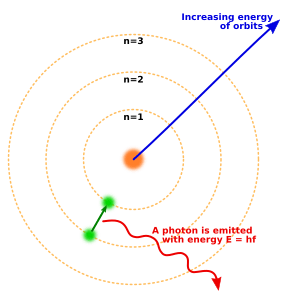The Niels Bohr model of the atom. Three electron shells about a nucleus, with an electron moving from the second to the first level and releasing a photon.

Electrons have the smallest electrical charge. This electrical charge equals the charge of a proton, but has the opposite sign. For this reason, electrons are attracted by the protons of atomic nuclei and usually form atoms. An electron has a mass of about 1/1836 times a proton. One way to think about the location of electrons in an atom is to imagine that they orbit at fixed distances from the nucleus. This way, electrons in an atom exist in a number of electron shells surrounding the central nucleus. Each electron shell is given a number 1, 2, 3, and so on, starting from the one closest to the nucleus (the innermost shell). Each shell can hold up to a certain maximum number of electrons. The distribution of electrons in the various shells is called electronic arrangement (or electronic form or shape). Electronic arrangement can be shown by numbering or an electron diagram. (A different way to think about the location of electrons is to use quantum mechanics to calculate their atomic orbitals.)

The electron is one of a class of subatomic particles called leptons. The electron has a negative electric charge. The electron has another property, called spin. Its spin value is 1/2, which makes it a fermion.

While most electrons are found in atoms, others move independently in matter, or together as cathode rays in a vacuum. In some superconductors, electrons move in pairs. When electrons flow, this flow is called electricity, or an electric current.

An object can be described as 'negatively charged' if there are more electrons than protons in an object, or 'positively charged' when there are more protons than electrons. Electrons can move from one object to another when touched. They may be attracted to another object with opposite charge, or repelled when they both have the same charge. When an object is 'grounded', electrons from the charged object go into the ground, making the object neutral. This is what lightning rods ( lightning conductors ) do.

### Chemical reactions

Electrons in their shells round an atom are the basis of chemical reactions. Complete outer shells, with maximum electrons, are less reactive. Outer shells with less than maximum electrons are reactive. The number of electrons in atoms is the underlying basis of the chemical periodic table.

### Measurement

Electric charge can be directly measured with a device called an electrometer. Electric current can be directly measured with a galvanometer. The measurement given off by a galvanometer is different from the measurement given off by an electrometer. Today laboratory instruments are capable of containing and observing individual electrons.

#### 'Seeing' an electron

In laboratory conditions, the interactions of individual electrons can be observed by means of particle detectors, which allow measurement of specific properties such as energy, spin and charge. In one instance a Penning trap was used to contain a single electron for 10 months. The magnetic moment of the electron was measured to a precision of eleven digits, which, in 1980, was a greater accuracy than for any other physical constant.

The first video images of an electron's energy distribution were captured by a team at Lund University in Sweden, February 2008. The scientists used extremely short flashes of light, called attosecond pulses, which allowed an electron's motion to be observed for the first time. The distribution of the electrons in solid materials can also be visualized.

### Anti-particle

The antiparticle of the electron is called a positron. This is identical to the electron, but carries electrical and other charges of the opposite sign. When an electron collides with a positron, they may scatter off each other or be totally annihilated, producing a pair (or more) of gamma ray photons.

### Classification

In the Standard Model of particle physics, electrons belong to the group of subatomic particles called leptons, which are believed to be fundamental or elementary particles. Electrons have the lowest mass of any charged lepton (or electrically charged particle of any type) and belong to the first-generation of fundamental particles. The second and third generation contain charged leptons, the muon and the tau, which are identical to the electron in charge, spin and interactions, but are more massive. Leptons differ from the other basic constituent of matter, the quarks, by their lack of strong interaction. All members of the lepton group are fermions, because they all have half-odd integer spin; the electron has spin 12.

### Quantum properties

As with all particles, electrons can act as waves. This is called the wave–particle duality and can be demonstrated using the double-slit experiment. The wave-like nature of the electron allows it to pass through two parallel slits simultaneously, rather than just one slit as would be the case for a classical particle. In quantum mechanics, the wave-like property of one particle can be described mathematically as a complex-valued function, the wave function, commonly denoted by the Greek letter psi (ψ). When the absolute value of this function is squared, it gives the probability that a particle will be observed near a location—a probability density.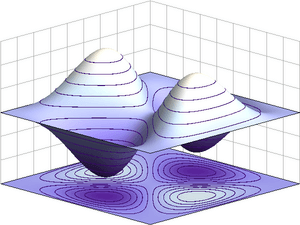Example of an antisymmetric wave function for a quantum state of two identical fermions in a 1-dimensional box. If the particles swap position, the wave function inverts its sign.

Electrons are identical particles because they cannot be distinguished from each other by their intrinsic physical properties. In quantum mechanics, this means that a pair of interacting electrons must be able to swap positions without an observable change to the state of the system. The wave function of fermions, including electrons, is antisymmetric, meaning that it changes sign when two electrons are swapped; that is, ψ(r1, r2) = −ψ(r2, r1), where the variables r1 and r2 correspond to the first and second electrons, respectively. Since the absolute value is not changed by a sign swap, this corresponds to equal probabilities. Bosons, such as the photon, have symmetric wave functions instead.

In the case of antisymmetry, solutions of the wave equation for interacting electrons result in a zero probability that each pair will occupy the same location or state. This is responsible for the Pauli exclusion principle, which precludes any two electrons from occupying the same quantum state. This principle explains many of the properties of electrons. For example, it causes groups of bound electrons to occupy different orbitals in an atom, rather than all overlapping each other in the same orbit.

### Virtual particles

Physicists believe that empty space may be continually creating pairs of virtual particles, such as a positron and electron, which rapidly annihilate each other shortly thereafter.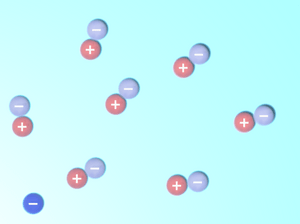A schematic depiction of virtual electron–positron pairs appearing at random near an electron (at lower left)

While an electron–positron virtual pair is in existence, the coulomb force from the ambient electric field surrounding an electron causes a created positron to be attracted to the original electron, while a created electron experiences a repulsion. This causes what is called vacuum polarization. In effect, the vacuum behaves like a medium having a dielectric permittivity more than unity. Thus the effective charge of an electron is actually smaller than its true value, and the charge decreases with increasing distance from the electron. This polarization was confirmed experimentally in 1997 using the Japanese TRISTAN particle accelerator. Virtual particles cause a comparable shielding effect for the mass of the electron.

The interaction with virtual particles also explains the small (about 0.1%) deviation of the intrinsic magnetic moment of the electron from the Bohr magneton (the anomalous magnetic moment). The extraordinarily precise agreement of this predicted difference with the experimentally determined value is viewed as one of the great achievements of quantum electrodynamics.

In classical physics, the angular momentum and magnetic moment of an object depend upon its physical dimensions. Hence, the concept of a dimensionless electron possessing these properties might seem inconsistent. The apparent paradox can be explained by the formation of virtual photons in the electric field generated by the electron. These photons cause the electron to shift about in a jittery fashion (known as zitterbewegung), which results in a net circular motion with precession. This motion produces both the spin and the magnetic moment of the electron. In atoms, this creation of virtual photons explains the Lamb shift observed in spectral lines.

### Atoms and molecules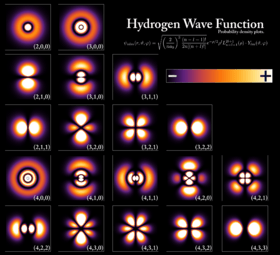Probability densities for the first few hydrogen atom orbitals, seen in cross-section. The energy level of a bound electron determines the orbital it occupies, and the color reflects the probability to find the electron at a given position.

An electron can be bound to the nucleus of an atom by the attractive Coulomb force. A system of one or more electrons bound to a nucleus is called an atom. If the number of electrons is different from the nucleus' electrical charge, such an atom is called an ion. The wave-like behavior of a bound electron is described by a function called an atomic orbital. Each orbital has its own set of quantum numbers such as energy, angular momentum and projection of angular momentum, and only a discrete set of these orbitals exist around the nucleus. According to the Pauli exclusion principle each orbital can be occupied by up to two electrons, which must differ in their spin quantum number.

Electrons can transfer between different orbitals by the emission or absorption of photons with an energy that matches the difference in potential. Other methods of orbital transfer include collisions with particles, such as electrons, and the Auger effect. In order to escape the atom, the energy of the electron must be increased above its binding energy to the atom. This occurs, for example, with the photoelectric effect, where an incident photon exceeding the atom's ionization energy is absorbed by the electron.

The orbital angular momentum of electrons is quantized. Because the electron is charged, it produces an orbital magnetic moment that is proportional to the angular momentum. The net magnetic moment of an atom is equal to the vector sum of orbital and spin magnetic moments of all electrons and the nucleus. The magnetic moment of the nucleus is negligible compared with that of the electrons. The magnetic moments of the electrons that occupy the same orbital (so called, paired electrons) cancel each other out.

The chemical bond between atoms occurs as a result of electromagnetic interactions, as described by the laws of quantum mechanics. The strongest bonds are formed by the sharing or transfer of electrons between atoms, allowing the formation of molecules. Within a molecule, electrons move under the influence of several nuclei, and occupy molecular orbitals; much as they can occupy atomic orbitals in isolated atoms. A fundamental factor in these molecular structures is the existence of electron pairs. These are electrons with opposed spins, allowing them to occupy the same molecular orbital without violating the Pauli exclusion principle (much like in atoms). Different molecular orbitals have different spatial distribution of the electron density. For instance, in bonded pairs (i.e. in the pairs that actually bind atoms together) electrons can be found with the maximal probability in a relatively small volume between the nuclei. On the contrary, in non-bonded pairs electrons are distributed in a large volume around nuclei.

### Conductivity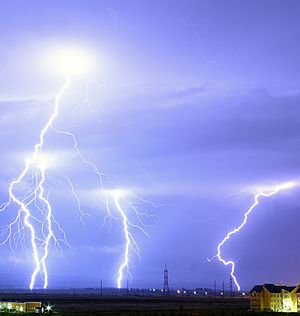A lightning discharge consists primarily of a flow of electrons. The electric potential needed for lightning may be generated by a triboelectric effect.

If a body has more or fewer electrons than are required to balance the positive charge of the nuclei, then that object has a net electric charge. When there is an excess of electrons, the object is said to be negatively charged. When there are fewer electrons than the number of protons in nuclei, the object is said to be positively charged. When the number of electrons and the number of protons are equal, their charges cancel each other and the object is said to be electrically neutral. A macroscopic body can develop an electric charge through rubbing, by the triboelectric effect.

Independent electrons moving in vacuum are termed free electrons. Electrons in metals also behave as if they were free. In reality the particles that are commonly termed electrons in metals and other solids are quasi-electrons—quasiparticles, which have the same electrical charge, spin and magnetic moment as real electrons but may have a different mass. When free electrons—both in vacuum and metals—move, they produce a net flow of charge called an electric current, which generates a magnetic field. Likewise a current can be created by a changing magnetic field. These interactions are described mathematically by Maxwell's equations.

At a given temperature, each material has an electrical conductivity that determines the value of electric current when an electric potential is applied. Examples of good conductors include metals such as copper and gold, whereas glass and Teflon are poor conductors. In any dielectric material, the electrons remain bound to their respective atoms and the material behaves as an insulator. Most semiconductors have a variable level of conductivity that lies between the extremes of conduction and insulation. On the other hand, metals have an electronic band structure containing partially filled electronic bands. The presence of such bands allows electrons in metals to behave as if they were free or delocalized electrons. These electrons are not associated with specific atoms, so when an electric field is applied, they are free to move like a gas (called Fermi gas) through the material much like free electrons.

Because of collisions between electrons and atoms, the drift velocity of electrons in a conductor is on the order of millimeters per second. However, the speed at which a change of current at one point in the material causes changes in currents in other parts of the material, the velocity of propagation, is typically about 75% of light speed. This occurs because electrical signals propagate as a wave, with the velocity dependent on the dielectric constant of the material.

Metals make relatively good conductors of heat, primarily because the delocalized electrons are free to transport thermal energy between atoms. However, unlike electrical conductivity, the thermal conductivity of a metal is nearly independent of temperature. This is expressed mathematically by the Wiedemann–Franz law, which states that the ratio of thermal conductivity to the electrical conductivity is proportional to the temperature. The thermal disorder in the metallic lattice increases the electrical resistivity of the material, producing a temperature dependence for electrical current.

When cooled below a point called the critical temperature, materials can undergo a phase transition in which they lose all resistivity to electrical current, in a process known as superconductivity. In BCS theory, this behavior is modeled by pairs of electrons entering a quantum state known as a Bose–Einstein condensate. These Cooper pairs have their motion coupled to nearby matter via lattice vibrations called phonons, thereby avoiding the collisions with atoms that normally create electrical resistance. (Cooper pairs have a radius of roughly 100 nm, so they can overlap each other.) However, the mechanism by which higher temperature superconductors operate remains uncertain.

Electrons inside conducting solids, which are quasi-particles themselves, when tightly confined at temperatures close to absolute zero, behave as though they had split into two other quasiparticles: spinons and holons. The former carries spin and magnetic moment, while the latter electrical charge.

### Motion and energy

According to Einstein's theory of special relativity, as an electron's speed approaches the speed of light, from an observer's point of view its relativistic mass increases, thereby making it more and more difficult to accelerate it from within the observer's frame of reference. The speed of an electron can approach, but never reach, the speed of light in a vacuum, c. However, when relativistic electrons—that is, electrons moving at a speed close to c—are injected into a dielectric medium such as water, where the local speed of light is significantly less than c, the electrons temporarily travel faster than light in the medium. As they interact with the medium, they generate a faint light called Cherenkov radiation.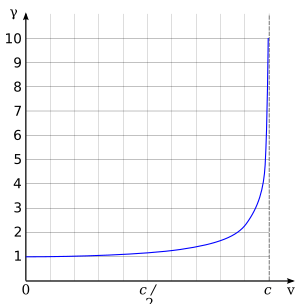Lorentz factor as a function of velocity. It starts at value 1 and goes to infinity as v approaches c.

Since an electron behaves as a wave, at a given velocity it has a characteristic de Broglie wavelength. This is given by λe = h/p where h is the Planck constant and p is the momentum. For the 51 GeV electron above, the wavelength is about 2.4×10−17 m, small enough to explore structures well below the size of an atomic nucleus.

## Related pagesElectron Facts for Kids. Kiddle Encyclopedia.